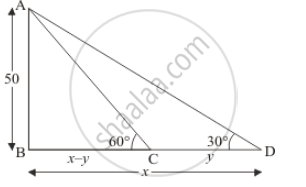# A Straight Highway Leads to the Foot of a Tower of Height 50 M. from the Top of the Tower, the Angles of Depression of Two Cars Standing on the Highway Are 30° and 60° Respectively. What is the Distance the Two Cars and How Far is Each Car from the Tower? - Mathematics

A straight highway leads to the foot of a tower of height 50 m. From the top of the tower, the angles of depression of two cars standing on the highway are 30° and 60° respectively. What is the distance the two cars and how far is each car from the tower?

#### Solution

Let AB be the height of tower 50 m and angle of depression from the top of the tower are 60° and 30° respectively at two observing Car C and D.

Let BD = x m, CD = y m and ∠ADB = 30°, ∠ACb = 60°

We have the corresponding figure as followsSo we use trigonometric ratios.

In a triangle ABD

=> tan D = (AB)/(BD)

=> tan 30° = 50/x

=> 1/sqrt3 = 50/x

=> x = 50sqrt3

Since x = 86.6

Again in a triangle ABC

=> tan C = (AB)/(BC)

=> tan 60° = 50/(x - y)

=> sqrt3 = 50/(x - y)

=> sqrtt3 xx 50sqrt3 - sqrt3y = 50

=> y = 57.67

Therefore x - y= 86.6 - 57.67

=> x - y = 28.93

Hence the distance of first car from tower is 86.6 m

And the distance of second car from tower is  57.67 m

And the distance between cars is 28.93 m

Is there an error in this question or solution?
Chapter 12: Trigonometry - Exercise 12.1 [Page 34]

#### APPEARS IN

RD Sharma Class 10 Maths
Chapter 12 Trigonometry
Exercise 12.1 | Q 63 | Page 34

Share